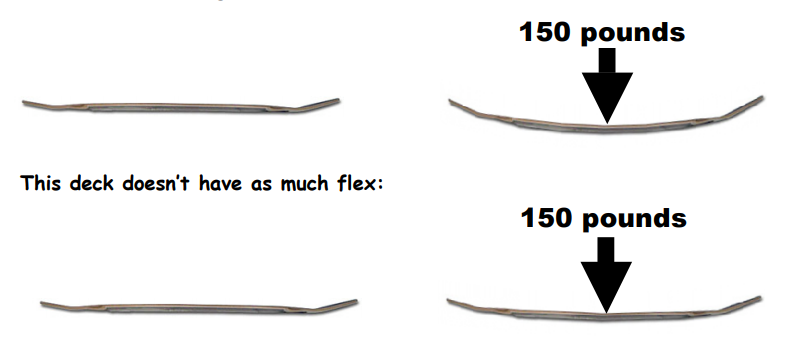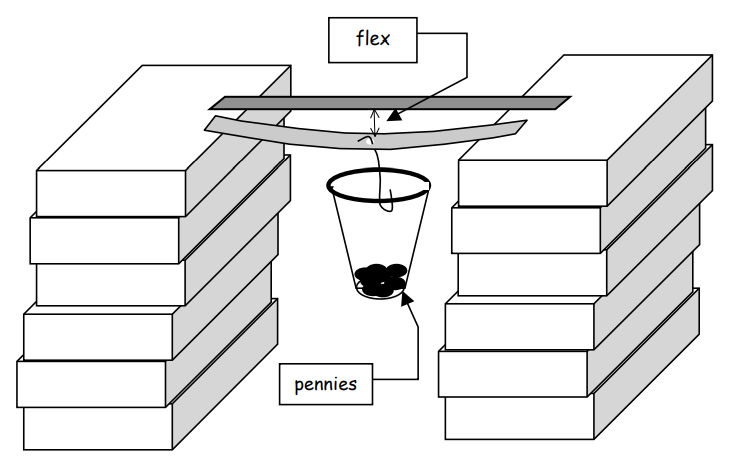Subject:
Mathematics

Common Core State Standard: 6.SP.2, 6.SP.3, 6.SP.5c,
Concepts:

• Ratio (explicit)
• Mean (explicit)
• Optional: Coordinates (explicit)
• Optional: Function (implicit)

Note: Note: “Explicit” refers to concepts which are named and discussed in the course of the lesson; “implicit” refers to concepts which students experience in the course of the lesson but which are not named and discussed.
Knowledge and Skills:

• Can describe a real world situation in terms of ratios.
• Can do operations with decimal numbers.
• Can find the mean of a set of data.
• Optional: Can plot a point in a two-dimensional coordinate system, given the coordinates.
• Optional: Can relate aspects of a graphical model to the real world situation which is being modeled.

Materials:

(for each team)
• Two rulers, one with a hole in the center
• One ruler with millimeter markings
• One heavy duty paper clip
• A plastic cup (the type with a rolled rim)
• 200 pennies

Lesson:

Procedure: This project should be done by students in teams of two to four.

Distribute the handout and review with students the description of the concept of “flex” on the first page (up to the point where the number activity instructions begin). Ask students who have experience with skateboards to explain why flex, and bounce, are important qualities of a deck.

Review the activity instructions, and as each group shows you that they understand the instructions, allow them to begin.

Circulate as students work and ensure that the concepts of “ratio” and “mean” are understood, and that the activity is being done correctly.

It is recommended that you ask students to do the final step individually, in order to assess each student’s individual understanding.

Optional Extension: Ask students, working in groups, to graph the ordered pairs of data that they have collected (number of pennies, flex), for each of the two rulers tested, on the same sheet of graph paper, and to explain what the graphs show. Discuss whether the amount of flex seems to depend on the number of pennies, how that dependence could be described, and in what ways that dependence is different for the two rulers tested.

### Skateboard Flex

One important quality of a skateboard is its “flex”—how far the deck will bend when weight is put on it, and how well it snaps back to its original shape.

The deck in this diagram has a lot of flex:(Actually, real skateboards have much less flex than either of these.)

The amount of flex that something has depends on what it is made of, and how it is made. Skateboard designers make their decks out of several thin layers of wood in order to give them the right amount of flex.

You can get an idea of how flex can be measured with this activity:

1. Get three rulers. One of them should have a hole in the center (such as plastic rulers usually have).

2. Put the ruler with a hole on two stacks of books at least one foot high, as shown in the diagram, and put the other rule right next to it. There should be exactly 1 inch of each end of the ruler on the books.

3. Get a plastic cup with a rolled rim, and punch a small hole just underneath the rim at one point. Put one end of a paper clip through that hole, and make a hook with the other end. Put the hook through the center hole in
the ruler, so that the cup is hanging between the stacks of books.

4. Add 25 pennies to the cup, and measure how far the center of the ruler is bent. Then add another 25 pennies, and measure again.

5. Make at least three more measurements with different amounts of pennies. Put your data in a table, like this:

 # of pennies Ruler flex (in millimeters) 25 3 50 6 75 10

6. For each measurement you made, divide the amount of ruler flex by the number of pennies in order to find the ratio of “flex per penny”, as a decimal. Find the mean of these ratios for all measurements.

7. Repeat the measurements with another ruler, one which is different from the first (for example, a wooden ruler, or a thicker plastic ruler).

8. On a separate sheet of paper, describe your results, and explain what the ratio of “flex per penny” tells you about the two rulers.